klaims,

ajo (bagger lingo), so here is my normal routine update. went outta town this weekend it all worked out pretty well. D\$ found this volvo fanatic on craigslist named tom. the trade was to give D\$ an engine (and install) for this 1970 140 volvo then give tom his current 1981 240 volvo. i went off on my bike and took some photos. got my freight train on. pretty fun...

stay grand,
_b
*dumpster funk windsurfing team.
**they worked from 8am-6am on this engine swap >THE PRO-CESS<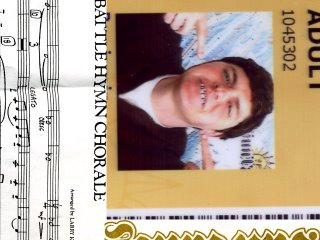shine shine hush hush ^^^^^^^^^^^^^^^^^^^^^^^^^^^^^^this mural is raw ^^^^^^^^^^^^^^^^^^^^^^^^^^^^^^^^^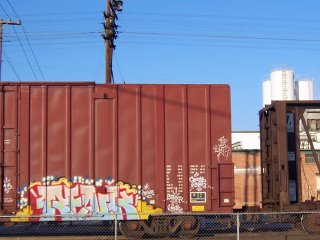renk sfr heading west @ 60 miles an hour ^^^^^^^^^^^^^^^^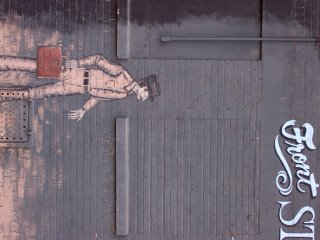don't front on front street^^^^^^^^^^^^^^^^^^^^^^^^^^^^don't fake: them boyz at war ^^^^^^^^^^^^^^^^^^^^^^^^^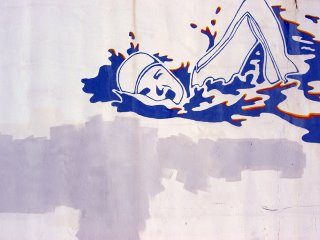don't swim if you don't know how ^^^^^^^^^^^^^^^^^^^^^^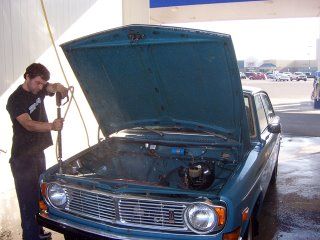sparkle motion ^^^^^^^^^^^^^^^^^^^^^^^^^^^^^^^^^^^logo check ^^^^^^^^^^^^^^^^^^^^^^^^^^^^^^^^^^^^^^goofy drawings: then put in my pocket ^^^^^^^^^^^^^^^^^^^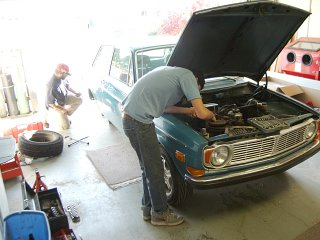i like volvos^^^^^^^^^^^^^^^^^^^^^^^^^^^^^^^^^^^^^dont play (act tuff with a burrito in your pocket) ^^^^^^^^^^^^